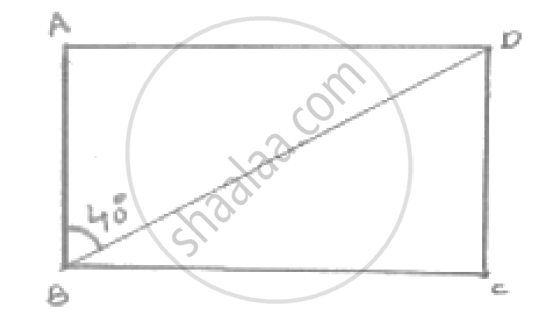Share

# Abcd is a Rectangle with ∠Abd = 40°. Determine ∠Dbc . - Mathematics

Course

#### Question

ABCD is a rectangle with ∠ABD = 40°. Determine ∠DBC .

#### SolutionWe have,

∠ABC = 90°

⇒ ∠ABD + ∠DBC = 90°      [ ∵ ∠ABD = 40°]

⇒ 40° + ∠DBC = 90°

∴∠DBC = 50°

Is there an error in this question or solution?

#### Video TutorialsVIEW ALL 

Solution Abcd is a Rectangle with ∠Abd = 40°. Determine ∠Dbc . Concept: Types of Quadrilaterals.
S